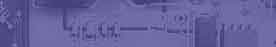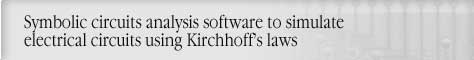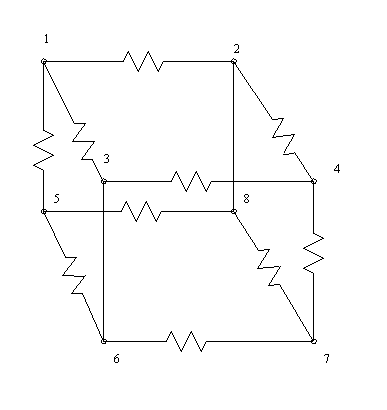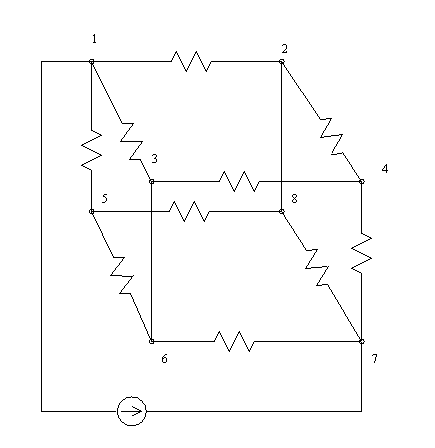Using circuit magic to calculate circuit resistancesCircuit analysis Tutorial
AKNM Circuit Magic- circuit analysis software

Using circuit magic to calculate circuit resistances

Circuit simplifications using star-to-delta, parallel and series transformations, seems pretty powerful. It allows one to obtain resistances a circuit that has a planar, mesh structure (the "window pane" structure). The term planar is used to describe circuits that can be drawn in a plane without the need for wires to cross one another. Circuit Magic can help you to obtain both planar and non-planar circuits resistances.

Sample circuit. Resistors resistances are different values (not simple, Try to simplify it using star-to-delta, parallel and series transformationsü)Step1. Connect node 1 and node 7 using branch with current source (current =1 amps)Step 2. Solve circuit using Node Voltage method

Step 2. Calculate circuit resistance using Ohms Law